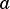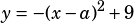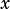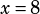In the equation below,is a positive integer constant.When the equation above is graphed in the-plane, one of the graph's-intercepts is at. What is the least possible value of?John Lockman says:

what subtracted from 8 when squared will give you 9 ( because it must be subtracted from 9), thus making y =0 must be 5infoanalysis says:

Looking at it right away you can see that what is in the parenthesis must be equal to +/-3 so that x intercept is when y =0
-(+/-3^2) =-9
-9+9 =0

8-5 = 3
8-11=-3